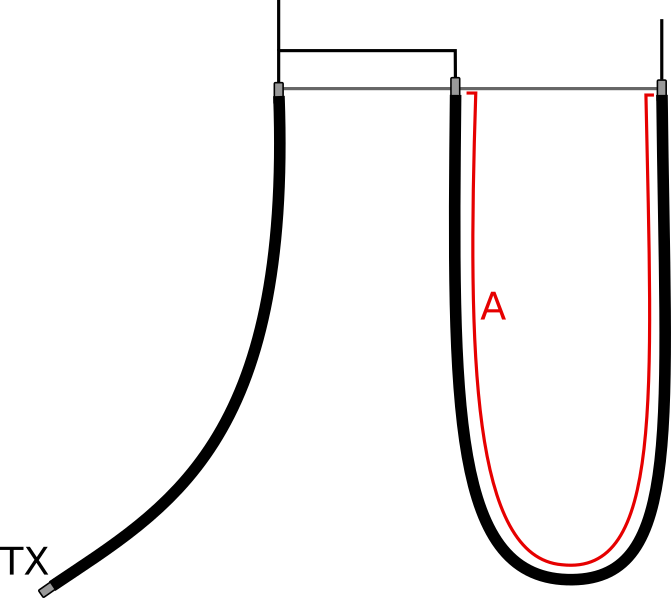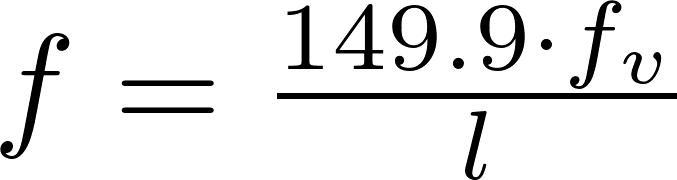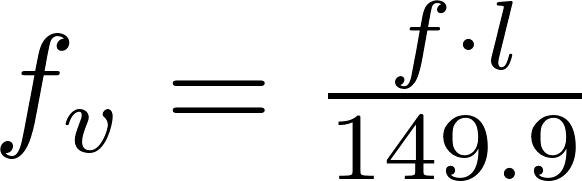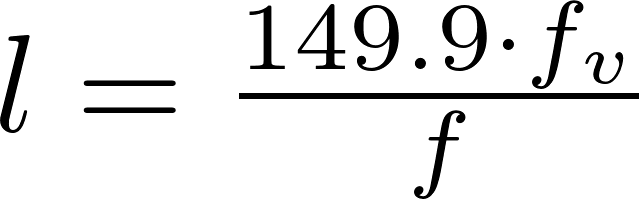# 4:1 Half-wave coax balun

The half-wave balun has two output. The first output is connected to the main cable, the second one is connected to the end of the half-wavelength coaxial cable.

### FormulasSchematicf is the frequency in megahertz (MHz).
f v is the velocity factor.
l is the symbol for length and is measured in meter (m).

### Calculator

Enter two values to calculate the third.